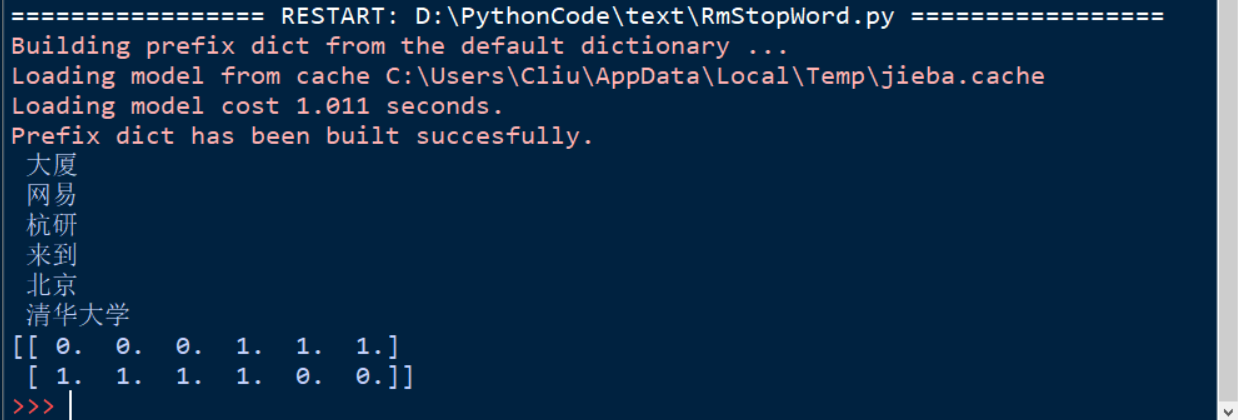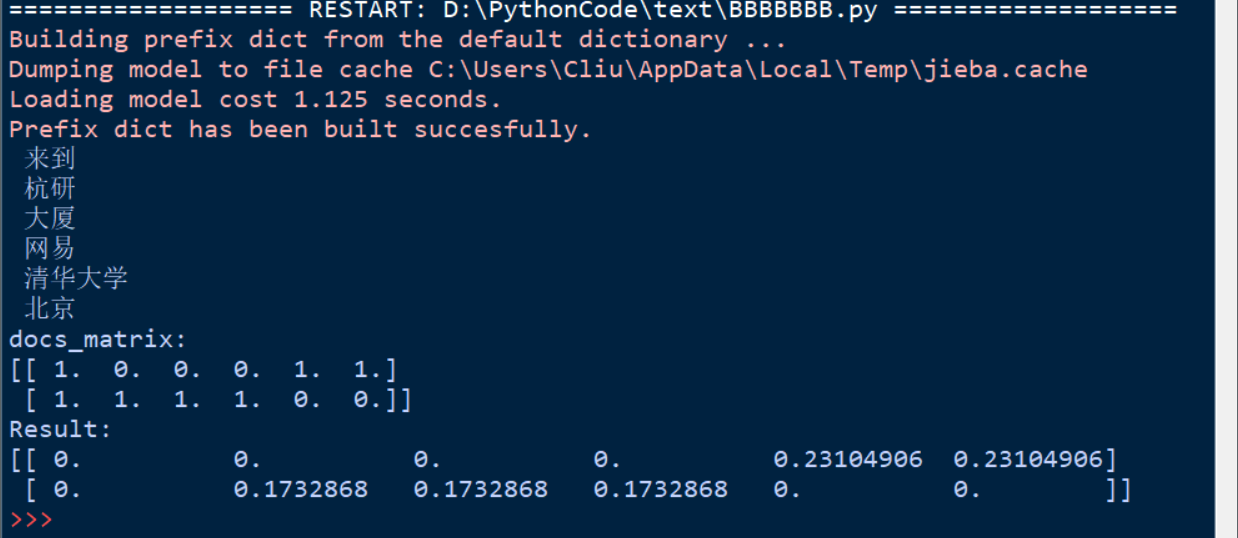# 概念：

Vector space model (or term vector model) is an algebraic model for representing text documents (and any objects, in general) as vectors of identifiers, such as, for example, index terms. It is used in information filtering, information retrieval, indexing and relevancy rankings. Its first use was in the SMART Information Retrieval System.

A document is represented as a vector. Each dimension corresponds to a separate term. If a term occurs in the document, its value in the vector is non-zero. Several different ways of computing these values, also known as (term) weights, have been developed. One of the best known schemes is tf-idf weighting.

The definition of term depends on the application. Typically terms are single words, keywords, or longer phrases. If the words are chosen to be the terms, the dimensionality of the vector is the number of words in the vocabulary (the number of distinct words occurring in the corpus).

1.我来到北京清华大学

2.他来到了网易杭研大厦# TF-IDF(term frequency–inverse document frequency)

TF-IDF是一种统计方法，用以评估一个词(term)对于一个文件集或者一个语料库中的一份文件的重要程度。一个词(term)的重要性随着它在文件中出现的次数成正比增加，但同时会随着它在语料库中出现的频率成反比下降。

TF-IDF的主要思想是：如果某个词或短语(term)在一篇文章中出现的频率TF高，并且在其他文章中很少出现，则认为此词或者短语(term)具有很好的类别区分能力，适合用来分类。如果包含词条term的文档越少，也就是n越小，则IDF越大，则说明词条term也具有很好的类别区分能力。

$tf_i,_j = \frac{n_i,_j}{\sum_k n_k,_j}$

TF公式解读：上式中分子是该词在文件中出现的次数，而分母则是该词在文件中出现的词数之和。

$idf(t,D) = log(\frac{N}{\lvert {d \in D, t \in d}\rvert})$

IDF公式解读：

|D|：语料库中文件的总数